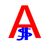# How to delete the empty dataset from gdb

648
4
03-31-2018 03:38 AMOccasional Contributor

Hi Dear,

Attached gdb here.How to delete the empty dataset from gdb in this gdb data available in HVAC, Fire alarm, Audiovisual, Fire Protection dataset rest of datasets empty for information any python code available plz help.

Thanks

Santhosh

Tags (2)
2 Solutions

Accepted SolutionsbyEsri Esteemed Contributor

To show you how you can delete empty featureclasses, feature datasets and tables, see the code below:

``````def main():
import arcpy
import os

fgdb = r'C:\GeoNet\DeleteEmpty\SSUC_FGDB_V3.gdb'

# list feature datasets
arcpy.env.workspace = fgdb
fdss = arcpy.ListDatasets()
fdss.append('')

# loop through feature datasets to delete empty ones
print("Delete empty featureclasses")
for fds in fdss:
print("Processing FDS: {}".format(fds))
fcs = arcpy.ListFeatureClasses('*', None, fds)
# loop through featureclasses in feature dataset
for fc_name in fcs:
fc = os.path.join(fgdb, fds, fc_name)
count = GetCount(fc)
if count == 0:
# if fc is empty, delete it
print(" - Deleting: {}".format(fc_name))
arcpy.Delete_management(fc)
else:
print(" - Skip: {}".format(fc_name))

# delete empty feature datasets
print("Delete empty feature datasets")
fdss = arcpy.ListDatasets()
for fds in fdss:
print("Processing FDS: {}".format(fds))
fcs = arcpy.ListFeatureClasses('*', None, fds)
if len(fcs) == 0:
print(" - Delete FDS {}".format(fds))
arcpy.Delete_management(fds)
else:
print(" - Keep FDS {}".format(fds))

# delete empty tables
print("Delete empty tables")
tbls = arcpy.ListTables()
for tbl_name in tbls:
tbl = os.path.join(fgdb, tbl_name)
count = GetCount(tbl)
if count == 0:
# if tbl is empty, delete it
print(" - Deleting: {}".format(tbl_name))
arcpy.Delete_management(tbl)
else:
print(" - Skip: {}".format(tbl_name))

def GetCount(fc):
return int(arcpy.GetCount_management(fc).getOutput(0))

if __name__ == '__main__':
main()‍‍‍‍‍‍‍‍‍‍‍‍‍‍‍‍‍‍‍‍‍‍‍‍‍‍‍‍‍‍‍‍‍‍‍‍‍‍‍‍‍‍‍‍‍‍‍‍‍‍‍‍‍‍‍‍‍‍``````byEsri Esteemed Contributor

You're welcome, I'm glad it worked.

4 RepliesbyMVP Legendary Contributor

Not sure what you mean by wanting 'arcpy'.

Through arcpy, you implement the code snippet to match your case using Delete_managementbyEsri Esteemed Contributor

To show you how you can delete empty featureclasses, feature datasets and tables, see the code below:

``````def main():
import arcpy
import os

fgdb = r'C:\GeoNet\DeleteEmpty\SSUC_FGDB_V3.gdb'

# list feature datasets
arcpy.env.workspace = fgdb
fdss = arcpy.ListDatasets()
fdss.append('')

# loop through feature datasets to delete empty ones
print("Delete empty featureclasses")
for fds in fdss:
print("Processing FDS: {}".format(fds))
fcs = arcpy.ListFeatureClasses('*', None, fds)
# loop through featureclasses in feature dataset
for fc_name in fcs:
fc = os.path.join(fgdb, fds, fc_name)
count = GetCount(fc)
if count == 0:
# if fc is empty, delete it
print(" - Deleting: {}".format(fc_name))
arcpy.Delete_management(fc)
else:
print(" - Skip: {}".format(fc_name))

# delete empty feature datasets
print("Delete empty feature datasets")
fdss = arcpy.ListDatasets()
for fds in fdss:
print("Processing FDS: {}".format(fds))
fcs = arcpy.ListFeatureClasses('*', None, fds)
if len(fcs) == 0:
print(" - Delete FDS {}".format(fds))
arcpy.Delete_management(fds)
else:
print(" - Keep FDS {}".format(fds))

# delete empty tables
print("Delete empty tables")
tbls = arcpy.ListTables()
for tbl_name in tbls:
tbl = os.path.join(fgdb, tbl_name)
count = GetCount(tbl)
if count == 0:
# if tbl is empty, delete it
print(" - Deleting: {}".format(tbl_name))
arcpy.Delete_management(tbl)
else:
print(" - Skip: {}".format(tbl_name))

def GetCount(fc):
return int(arcpy.GetCount_management(fc).getOutput(0))

if __name__ == '__main__':
main()‍‍‍‍‍‍‍‍‍‍‍‍‍‍‍‍‍‍‍‍‍‍‍‍‍‍‍‍‍‍‍‍‍‍‍‍‍‍‍‍‍‍‍‍‍‍‍‍‍‍‍‍‍‍‍‍‍‍``````Occasional Contributor

Xander Bakker ,

Thank you So much .

Thanks

SanthoshbyEsri Esteemed Contributor

You're welcome, I'm glad it worked.